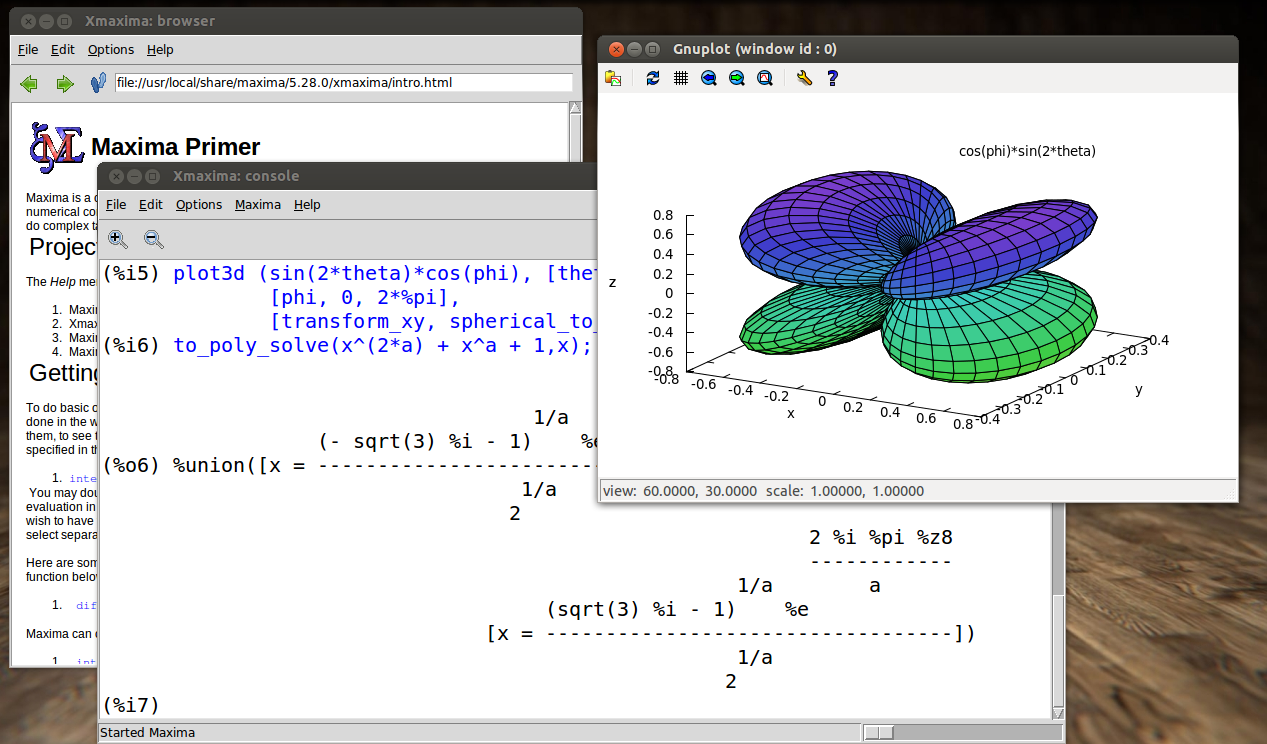News » #Maxima added to FreeSMUG directory

Posted on 30 May 2016 07:42Maxima Computer Algebra System written in Common Lisp added to Dev/Utils directory.

Maxima is a system for the manipulation of symbolic and numerical expressions, including differentiation, integration, Taylor series, Laplace transforms, ordinary differential equations, systems of linear equations, polynomials, sets, lists, vectors, matrices and tensors. Maxima yields high precision numerical results by using exact fractions, arbitrary-precision integers and variable-precision floating-point numbers. Maxima can plot functions and data in two and three dimensions.

Maxima is a descendant of Macsyma, the legendary computer algebra system developed in the late 1960s at the Massachusetts Institute of Technology. It is the only system based on that effort still publicly available and with an active user community, thanks to its open source nature. Macsyma was revolutionary in its day, and many later systems, such as Maple and Mathematica, were inspired by it.

Maxima is a computer algebra system comparable to commercial systems like Mathematica and Maple. It emphasizes symbolic mathematical computation: algebra, trigonometry, calculus, and much more.
For example, Maxima solves x^2-r*x-s^2-r*s=0 giving the symbolic results [x=r+s, x=-s].

Maxima can calculate with exact integers and fractions, native floating-point and high-precision big floats.
Maxima has user-friendly front-ends, an on-line manual, plotting commands, and numerical libraries. Users can write programs in its native programming language, and many have contributed useful packages in a variety of areas over the decades.

## Features

• Specialized in symbolic operations but offering numerical capabilities too.
• Can be accessed programmatically and extended, as the underlying Lisp can be called from it.
• Complete programming language with ALGOL-like syntax but Lisp-like semantics.
• Arbitrary-precision integers.
• Rational numbers of sizes limited only by machine memory.
• Arbitrarily large floating-point numbers ("bfloats").